Author: Oscar Cronquist Article last updated on October 19, 2021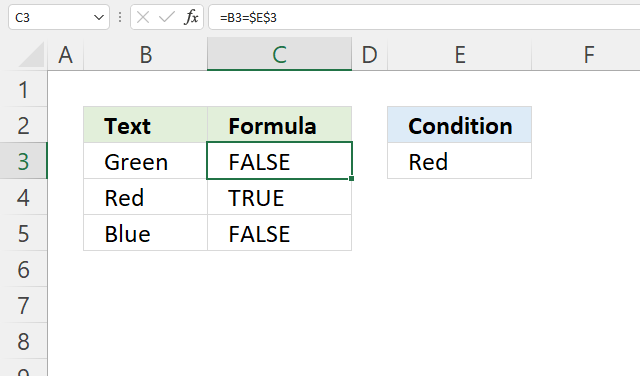This article demonstrates different formulas based on if a cell contains a given text.

Formula in cell C3:

=B3=\$E\$3

The formula shown in the image above in cell C3 returns TRUE or FALSE based on a comparison between cell B3 and cell E3. The equal sign is a logical operator and returns boolean value TRUE or FALSE, it is not case sensitive.

## 1. If cell contains partial text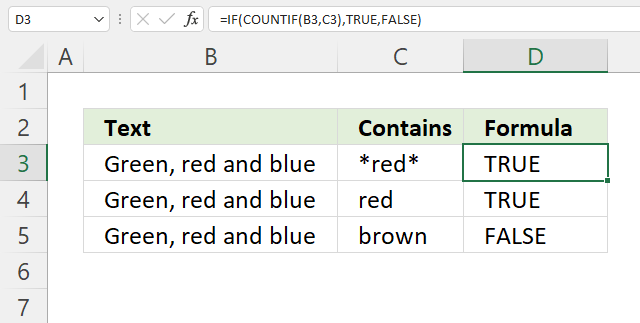The easiest way to check if a cell partially contains a specific text string is, in my opinion, the IF and COUNTIF function combined. The COUNTIF function allows you to count how many times a text string exists in a cell range.

Formula in cell D3:

=IF(COUNTIF(B3, C3), TRUE, FALSE)

The asterisk characters let you perform a wildcard match meaning that it matches any sequence of characters. Adding a beginning and ending asterisk to a text string allows you to check if a cell value contains a specific text string.

### 1.1 Explaining formula in cell D3

#### Step 1 - Check if cell contains text condition

You can't use asterisks with the equal sign to check if a cell contains a given text, however, the COUNTIF function can do that.

The COUNTIF function calculates the number of cells that is equal to a condition.

COUNTIF(rangecriteria)

COUNTIF(B3, C3)

becomes

COUNTIF("Green, red and blue","*red*")

and returns 1.

#### Step 2 - Evaluate IF function

The IF function returns one value if the logical test is TRUE and another value if the logical test is FALSE.

IF(logical_test, [value_if_true], [value_if_false])

IF(COUNTIF(B3, C3), TRUE, FALSE)

becomes

IF(1, TRUE, FALSE)

The logical_test argument requires boolean values or their numerical equivalents. FALSE = 0 (zero), TRUE any number except zero.

### 1.2 If cell contains partial text - hardcoded asterisks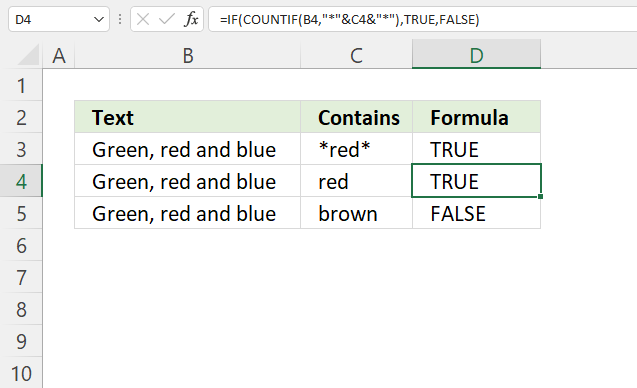Formula in cell D4:

=IF(COUNTIF(B4, "*"&C4&"*"), TRUE, FALSE)

You don't have to add asterisks manually to your cell, you can easily build a formula that adds the asterisks automatically, demonstrated in cell D4. The ampersand & character lets you append the asterisks to the text string you want to use.

Note, if a text string is found twice in the same cell the COUNTIF function only returns 1. It counts cells not text strings.

### 1. Alternative function - SEARCH function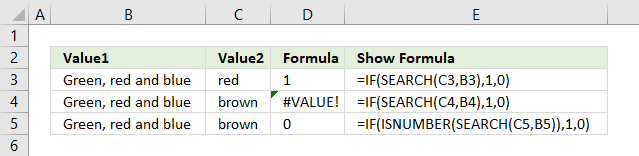The SEARCH function returns the position of the character at which a specific text string is found. Luckily the IF function accepts any number as TRUE except 0 (zero), however, the SEARCH function returns an error #VALUE! if it can't find the text string.

Formula in cell D5:

=IF(ISNUMBER(SEARCH(C5, B5)),1,0)

To avoid the error value I use the ISNUMBER function that returns TRUE if a number and FALSE if anything else, also formula errors.

## 2. If cell contains text then return value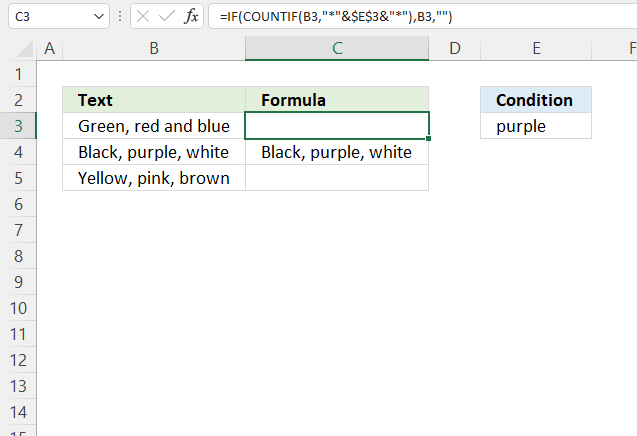The formula in cell C3 checks if cell B3 contains the condition specified in cell E3. It returns the value if TRUE and a blank cell if FALSE.

Formula in cell C3:

=IF(COUNTIF(B3, "*"&\$E\$3&"*"), B3, "")

### 2.1 Explaining formula in cell C3

#### Step 1 - Concatenate strings

The asterisk is a wildcard character that matches 0 (zero) to any number of characters. The ampersand character concatenates strings in an Excel formula.

"*"&\$E\$3&"*"

becomes

"*"&"purple"&"*"

and returns "*purple*".

#### Step 2 - Check if cell contains string

The COUNTIF function calculates the number of cells that is equal to a condition.

COUNTIF(rangecriteria)

COUNTIF(B3, "*"&\$E\$3&"*")

becomes

COUNTIF("Green, red and blue", "*purple*")

#### Step 3 - Return value if TRUE

The IF function returns one value if the logical test is TRUE and another value if the logical test is FALSE.

IF(logical_test, [value_if_true], [value_if_false])

IF(COUNTIF(B3, "*"&\$E\$3&"*"), B3, "")

becomes

IF(0, B3, "")

and returns "" in cell C3.

## 3. If cell contains text then add text from another cell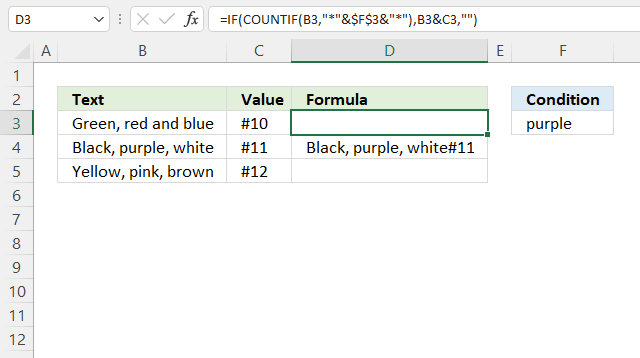The formula in cell C3 checks if cell B3 contains the condition specified in cell E3. It returns the value concatenated with a value on the same row from column if TRUE and a blank cell if FALSE.

Formula in cell C3:

=IF(COUNTIF(B3, "*"&\$F\$3&"*"), B3&C3, "")

### 3.1 Explaining formula in cell C4

#### Step 1 - Concatenate strings

The asterisk is a wildcard character that matches 0 (zero) to any number of characters. The ampersand character concatenates strings in an Excel formula.

"*"&\$E\$3&"*"

becomes

"*"&"purple"&"*"

and returns "*purple*".

#### Step 2 - Check if cell contains string

The COUNTIF function calculates the number of cells that is equal to a condition.

COUNTIF(rangecriteria)

COUNTIF(B4, "*"&\$E\$3&"*")

becomes

COUNTIF("Black, purple, white", "*purple*")

and returns 1. Purple is found in cell B4.

#### Step 3 - Return concatenated value if TRUE

The IF function returns one value if the logical test is TRUE and another value if the logical test is FALSE.

IF(logical_test, [value_if_true], [value_if_false])

IF(COUNTIF(B4, "*"&\$E\$3&"*"), B4&C4, "")

becomes

IF(0, B4&C4, "")

becomes

IF(0, "Black, purple, white"&"#11", "")

and returns "Black, purple, white#11" in cell D4.

## 4. If cell contains text then sum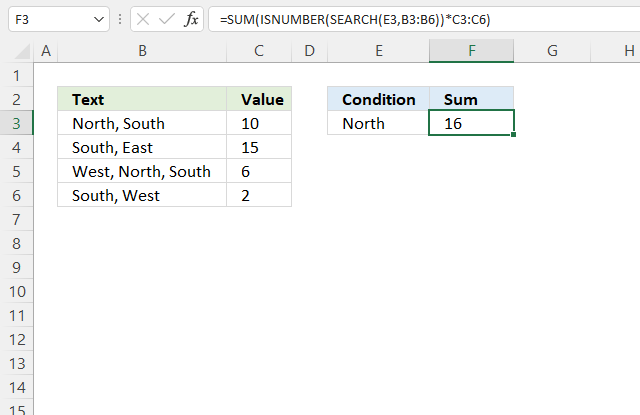The formula in cell F3 checks if cells in column B contain the condition specified in cell E3 and sums the corresponding values in column C.

Formula in cell C3:

=SUM(ISNUMBER(SEARCH(E3, B3:B6))*C3:C6)

### 4.1 Explaining formula in cell C4

#### Step 1 - Search for string

The SEARCH function returns a number representing the position of character at which a specific text string is found reading left to right. It is not a case-sensitive search.

SEARCH(find_text,within_text, [start_num])

SEARCH(E3, B3:B6)

becomes

SEARCH("North", {"North, South"; "South, East"; "West, North, South"; "South, West"})

and returns {1; #VALUE!; 7; #VALUE!}.

#### Step 2 - Check if value in array is an error

The ISNUMBER function checks if a value is a number, returns TRUE or FALSE.

ISNUMBER(SEARCH(E3, B3:B6))

becomes

ISNUMBER({1; #VALUE!; 7; #VALUE!})

and returns {TRUE; FALSE; TRUE; FALSE}.

#### Step 3 - Multiply corresponding numbers

The asterisk lets you multiply numbers in an Excel formula. We are multiplying boolean values in this example.

ISNUMBER(SEARCH(E3, B3:B6))*C3:C6

becomes

{TRUE; FALSE; TRUE; FALSE}*{10; 15; 6; 2}

TRUE equals 1 and FALSE equals 0 (zero).

{TRUE; FALSE; TRUE; FALSE}*{10; 15; 6; 2}

and returns {10; 0; 6; 0}.

#### Step 4 - Sum numbers

The SUM function adds numbers and returns a total.

SUM(ISNUMBER(SEARCH(E3, B3:B6))*C3:C6)

becomes

SUM({10; 0; 6; 0})

and returns 16 in cell F3.

## 5. If cell contains text add 1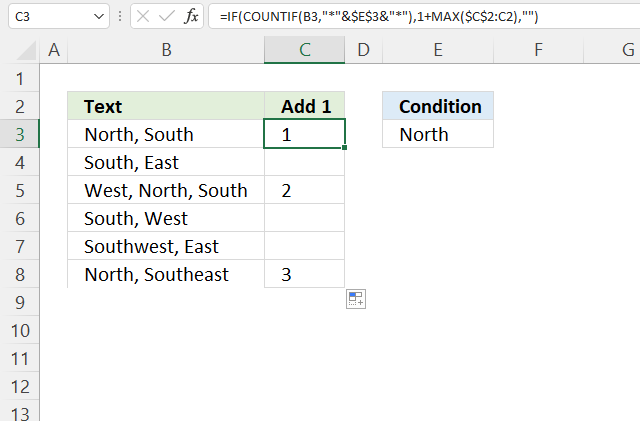The formula in cell C3 counts cells containing the given text string in cell E3.

Formula in cell C3:

=IF(COUNTIF(B3,"*"&\$E\$3&"*"),1+MAX(\$C\$2:C2),"")

### 5.1 Explaining formula in cell C3

#### Step 1 - Concatenate strings

The asterisk is a wildcard character that matches 0 (zero) to any number of characters. The ampersand character concatenates strings in an Excel formula.

"*"&\$E\$3&"*"

becomes

"*"&"North"&"*"

and returns "*North*".

#### Step 2 - Check if cell contains given string

The COUNTIF function calculates the number of cells that is equal to a condition.

COUNTIF(rangecriteria)

COUNTIF(B3,"*"&\$E\$3&"*")

becomes

COUNTIF("North, South", "*North*")

and returns 1.

#### Step 3 - Calculate count

The MAX function returns the largest number from a cell range or array.

Reference \$C\$2:C2 contains both absolute and relative cell references which makes it grow when the cell is copied to cells below.

1+MAX(\$C\$2:C2)

becomes

1+0

and returns 1.

#### Step 4 - Show count if corresponding cell contains given string

The IF function returns one value if the logical test is TRUE and another value if the logical test is FALSE.

IF(logical_test, [value_if_true], [value_if_false])

IF(COUNTIF(B3,"*"&\$E\$3&"*"),1+MAX(\$C\$2:C2),"")

becomes

IF(C1,1+MAX(\$C\$2:C2),"")

becomes

IF(C1, 1, "")

and returns 1.